Signin or Join to use this module.

Rhythm Analysis Method - 314 - Junctional Dysrhythmias.

Intro

The five steps of rhythm analysis will be followed when analyzing any rhythm strip. Analyze each tracing in the following order.

• Rhythm Regularity
• Heart Rate
• P wave morphology
• P R interval or PRi
• QRS complex duration and morphology

Step 1

Rhythm Regularity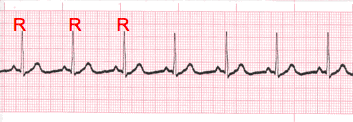• Carefully measure from the tip of one R wave to the next, from the beginning to the end of the tracing.
• A rhythm is considered “regular or constant” when the distance apart is either the same or varies by 1 ½ small boxes or less from one R wave to the next R wave.

Step 2

Heart Rate Regular (Constant) Rhythms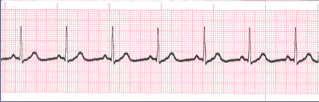• The heart rate determination technique used will be the 1500 technique.
• Starting at the beginning of the tracing through the end, measure from one R wave to the next R wave (ventricular assessment), then P wave to P wave (atrial assessment), then count the number of small boxes between each and divide that number into 1500. This technique will give you the most accurate heart rate when analyzing regular heart rhythms. You may include ½ of a small box i.e. 1500/37.5 = 40 bpm (don’t forget to round up or down if a portion of a beat is included in the answer).

Step 2 (Cont)

Heart Rate - Irregular Rhythms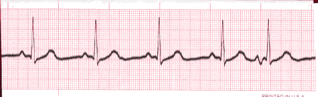• If the rhythm varies by two small boxes or more, the rhythm is considered “irregular”.
• The heart rate determination technique used for irregular rhythms will be the “six-second technique”.
• Simply count the number of cardiac complexes in six seconds and multiply by ten.

Step 3

P wave Morphology (shape)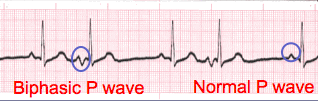• Lead II is most commonly referenced in cardiac monitoring
• In this training module, lead two will specifically referenced unless otherwise specified.

Step 4

PR interval (PRi)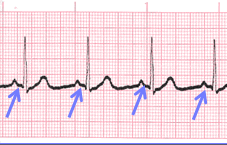Constant PR Interval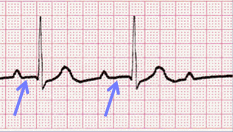Variable PR Interval
• Measurement of the PR interval reflects the amount of time from the beginning of atrial depolarization to the beginning of ventricular depolarization.
• Plainly stated, this measurement is from the beginning of the P wave to the beginning of the QRS complex.
• The normal range for PR interval is: 0.12 – 0.20 seconds (3 to 5 small boxes)
• It is important that you measure each PR interval on the rhythm strip.
• Some tracings do not have the same PRi measurement from one cardiac complex to the next. Sometimes there is a prolonging pattern, sometimes not.
• If the PR intervals are variable, report them as variable, but note if a pattern is present or not.

Step 5

QRS complex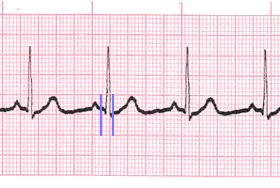• QRS represents ventricular depolarization.
• It is very important to analyze each QRS complex on the tracing and report the duration measurement and describe the shape (including any changes in shape).
• As discussed in step 3, when referring to P waves, remember changes in the shape of the waveform can indicate the locus of stimulation has changed or a different conduction pathway was followed. It is no different when analyzing the QRS complex. The difference is that in step 3, we were looking at atrial activity. Now we are looking at ventricular activity.
• Measure from the beginning to the end of ventricular depolarization.
• The normal duration of the QRS complex is: 0.06 – 0.10 second